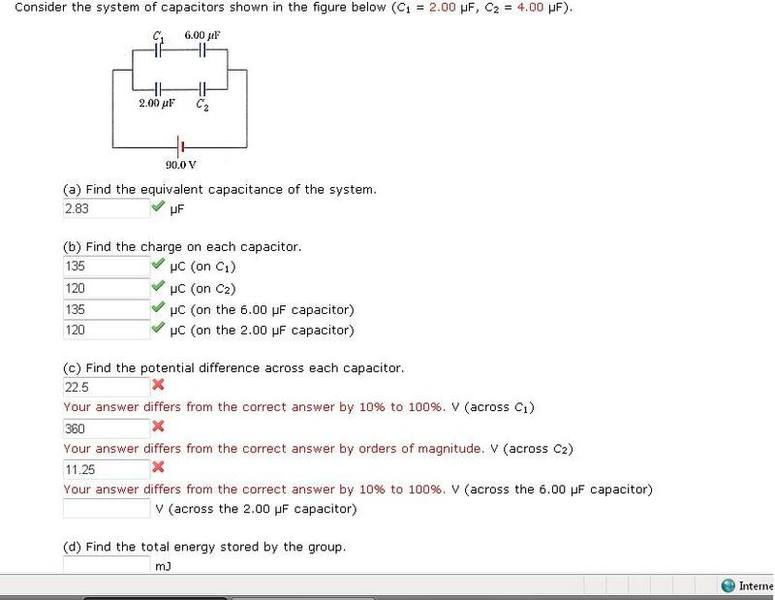# Potential Difference across Capacitor.

## Homework Statement## Homework Equations

Not sure which equations to use but I know that we'll have to use the answers from part a and b to answer the questions.

## The Attempt at a Solution

What I did was that I said that C1 and the 6 microfarads are parallel to C2 and the 2 microfarads capacitor and said that the voltage would just be halved. Really confused on what to do.

remember that voltage is not 'split up' to components in parallel, this only occurs to components in series, which is the second half of the problem

remember that voltage is not 'split up' to components in parralel, both the top & the bottom will receive 90V (separateley) and the 90 is shared between the two capacitors.

hmmm thanks that helps to clarify that point. So its 90 volts to C1 and the 6 microfarads capacitor and to the C2 and 2 microfarads capacitor. What equation do I need to use? I have a couple here. Looking at PE=(.5)(C)(deltaV^2) is that the right one? I think the main problem here is that I dont know what equation to use or how to look at the problem.

hmm i'm afraid not, that's the equation for working out the energy stored in the capacitor, so you'll need it later, however

V = Q / C voltage = charge / capacitance , you know the Q & C for each capacitor, so try to work out V

hmm i'm afraid not, that's the equation for working out the energy stored in the capacitor, so you'll need it later, however

V = Q / C voltage = charge / capacitance , you know the Q & C for each capacitor, so try to work out V

hmmm....Im still getting some wrong answers. So lets say for C2, I know the charge on that capacitor is 120 microCouloumbs. Im not sure what the Capacitance for it is. Would the capacitance for C2 be the sum of (1/C1)+(1/C2)=(1/Ctot)? so it would be that V=120/1.5?

Just figured out this part. Now onto the total energy stored by group?

the equation used would be PE=(.5)(C)(deltaV^2) ?

Last edited:
correcto, but look

let's take C1, you worked out earlier that charge was 120 * 10^-6
and you also know that it's capacitance is 2 * 10^-6 -it tells you on the top of the picture!

so now you have Q and C, and V = Q / C , they seem to work, try them again

Problem solved!

Thanks a million.